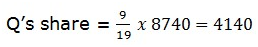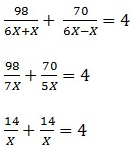# NIACL AO Prelims – Quantitative Aptitude Questions 2021 Day- 02

Dear Aspirants, Our IBPS Guide team is providing new series of Quantitative Aptitude Questions for NIACL AO Prelims 2021 so the aspirants can practice it on a daily basis. These questions are framed by our skilled experts after understanding your needs thoroughly. Aspirants can practice these new series questions daily to familiarize with the exact exam pattern and make your preparation effective.

### Start Quiz

Partnership

1) Rekha and Tarun started the business with the investment of Rs.(x – 2000) and (x + 1000) respectively. At the end of the year, Rekha received the profit is 33(1/3)% of the total profit of the business. What is the initial investment of Tarun?

A.Rs.5000

B.Rs.4000

C.Rs.6000

D.Rs.8000

E.Rs.7000

Boat and stream

2) The boat covers 120 km downstream in 6 hours and the same boat covers 45 km upstream in 4.5 hours. Find the speed of stream?

A.3 kmph

B.4 kmph

C.10 kmph

D.5 kmph

E.6 kmph

SI AND CI

3) Geetha invests Rs.x in simple interest scheme at the rate of 18% per annum for 3 years and Kanna invests Rs.(x – 2000) on compound interest scheme at the rate of 10% per annum for 3 years. If the interest received by Geetha is 72% of the amount invests by Kanna, find the compound interest received by Kanna?

A.Rs.1986

B.Rs.2016

C.Rs.1996

D.Rs.2040

E.None of these

Ages

4) Ratio of the ages of Prabha and Banu is 5:4 and Prabha is 20 years elder than Dina. The ratio of the ages of Dina and Renu is 1:3 and Banu is 8 years elder than Esai. If the present age of Banu is 24 years, then find the average ages of Prabha, Banu, Dina, Renu and Esai?

A.20 years

B.22 years

C.24 years

D.26 years

E.28 years

Percentage

5) Ratio of the income of Anil and Nila is 5:6 and the ratio of the expenditure of Anil and Nila is 4:5. If the savings of Anil and Nila is same and the difference between the expenditure of Nila and Anil is Rs.4000, then find the income of Anil?

A.Rs.10000

B.Rs.15000

C.Rs.20000

D.Rs.25000

E.Rs.30000

Percentage

6) The monthly expenditure of Amuthan is 80% of his monthly salary and the ratio of the monthly salary and expenditure of Bharathi is 4:3. If the savings of Amuthan and Bharathi is 5:3 and the monthly income of Amuthan is Rs.12500, then find the income of Bharathi?

A.Rs.5600

B.Rs.6000

C.Rs.6400

D.Rs.5200

E.Rs.4800

SI AND CI

7) A sum of amount invests in a compound interest scheme at 20% per annum for 2 years. If the interest is half yearly and the interest received from the CI scheme is Rs.1392.3, then find the sum?

A.Rs.3000

B.Rs.3600

C.Rs.4000

D.Rs.4500

E.Rs.4800

Average

8) The average weight of 30 employees in the company is 60 kg. If the weight of a manager is included, the average weight increased by 2 kg, then find the weight of the manager?

A.80 kg

B.88 kg

C.96 kg

D.102 kg

E.None of these

Partnership

9) P and Q started a business by investing Rs 12000 and Rs 18000 respectively. The time period for which P and Q invested was in the ratio of 5:3. If the annual profit received by both of them was Rs 8740, then what was Q’s share in the profit?

A.4140

B.3950

C.4550

D.5050

E.None of these

Boat and stream

10) Find the upstream speed of a boat, if the ratio of boat in still water to the speed of the stream is 6:1. It takes totally 4 hours by a boat to cover 98 km downstream and 70 km upstream.

A.35 kmph

B.49 kmph

C.98 kmph

D.70 kmph

E.None of these

### Try NIACL AO Online Practice Mock Test

Profit share of Rekha and Tarun = 33(1/3):66(2/3) = 1:2

(x – 2000)/(x + 1000) = 1/2

x + 1000 = 2x – 4000

x = 5000

Investment of Tarun = 5000 + 1000 = Rs.6000

Downstream speed = 120/6 = 20 kmph

Upstream speed = 45/4.5 = 10 kmph

Speed of stream = (20 – 10)/2 = 5 kmph

SI = P * R * N/100

CI = P * (1 + R/100)n – P

(x – 2000) * 72/100 = x * 18*3/100

4x – 8000 = 3x

x = 8000

CI received by Kanna = 6000 * (1 + 10/100)3 – 6000

= Rs.1986

Banu = 24 years

Esai = 24 – 8 = 16 years

Prabha = 5/4 * 24 = 30 years

Dina = 30 – 20 = 10 years

Renu = 3/1 * 10 = 30 years

Required average = (24 + 16 + 30 + 10 + 30)/5

= 22 years

Expenditure of Anil = 4 * 4000 = 16000

Expenditure of Nila = 5 * 4000 = 20000

(5x – 16000)/(6x – 20000) = 1/1

6x – 20000 = 5x – 16000

x = 4000

Income of Anil = 5 * 4000 = 20000

Expenditure of Amuthan = 80/100 * 12500 = 10000

Savings of Amuthan = 12500 – 10000 = 2500

Savings of Bharathi = 3/5 * 2500 = 1500

Income of Bharathi = 4/1 * 1500 = Rs.6000

The interest is half yearly = 20/2 = 10%

Half yearly time = 4 years

x * (1 + 10/100)4 – x = 1392.3

0.4641x = 1392.3

x = 3000

Let Weight of the manager = x

30 * 60 + x = 62 * 31

x = 122 kg

P                           Q

Ratio of Profit               12000 x 5     :        18000 x 3

10           :                9Let the speed of the boat in still water and that of the stream be 6X kmph and X kmph respectively.

According to question,X = 7

Upstream speed = 6X – X = 5X = 5 * 7 = 35 kmph